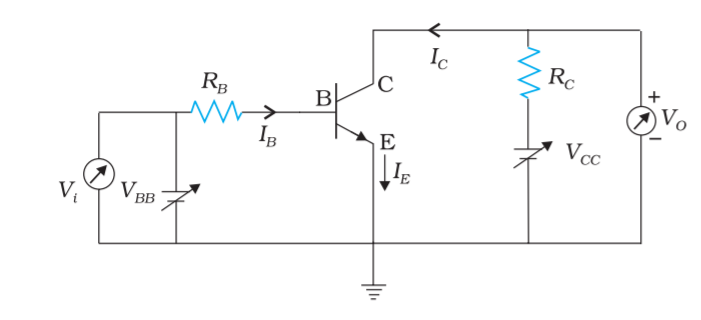Home/Class 12/Physics/

## QuestionPhysicsClass 12

In figure , the   $$V_{\mathit{BB}}\text{ supply can be varied from }0$$ V to $$5.0V$$ . The Si transistor has $$\beta _{\mathit{dc}}=250$$  and $$R_B=100k\Omega ,R_C=1KV_{\mathit{CC}}=5.0V$$  Assume that when the transistor is saturated, $$V_{\mathit{CE}}=0V$$  and $$V_{\mathit{BE}}=0.8\mathit{V.}$$  Find the ranges of $$V{_1}$$  for which the transistor is 'switched off and 'switched on'.Given at saturation $$V_{\mathit{CE}}=0V,V_{\mathit{BE}}=0.8V$$
$$V_{\mathit{CE}}=V_{\mathit{CC}}-I_CR_C$$
$$I_C=\frac{V_{\mathit{CC}}}{R_C}=\frac{5.0V}{1.0k\Omega} =5.0\mathit{mA}$$
Therefore $$I_B=\frac{I_C}{\beta} =\frac{5.0\mathit{mA}}{250}=20\mu A$$
The input voltage at which the transistor will go into saturation is given by $$V_{\mathit{IH}}=V_{\mathit{BB}}=I_BR_B+V_{\mathit{BE}}$$
$$=20\mu A\times 100k\Omega +0.8V=2.8V$$
The value of input voltage below which the transistor remains cutoff is given by $$V_{\mathit{IL}}=0.6V,V_{\mathit{IH}}=2.8V$$
Between $$0.0V$$  and $$0.6V,$$  the transistor will be in the 'switched off state. Between $$2.8V$$  and $$5.0V,$$  it will be in 'switched on' state.
Note that the transistor is in active state when $$I_B$$  varies from $$0.0\mathit{mA}$$  to $$20\mathit{mA}$$ . In this range, $$I_C=\beta I_B$$  is valid. In the saturation range, $$I_C{\leq}\beta I_B$$